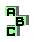POINT X(56)

 r141 tangential triangle, perspective, intangential triangle, incenter r142 point X(12), Gergonne point, circumcenter, Feuerbach point, incenter, orthocenter, centroid, Schiffler point r155 Mannheim circles, perspective r150 circumcircle, incircle, tangent circles, perspective r193 Schröder point, Weill point,Bevan-Schröder point, Bevan point, poristic triangles, point X(65), point X(55) d Bevan-Schröder point r516 Jenkins circles, excircles, isotomic points r547 intouch triangle, incircle, homothetic triangles r1156 point X(55), inconic, perspector, Feuerbach point, isogonal points r1327 circumcircle, incenter r1440 circumcevian triangle, isogonal points, incenter, homothetic triangles r1541 incircle r1638 circumcevian triangle, perspective, incenter, circumcenter r1752 altitude feet, radical center r1833 isogonal points, incenter, circumcircle, Mannheim circles, Spieker point